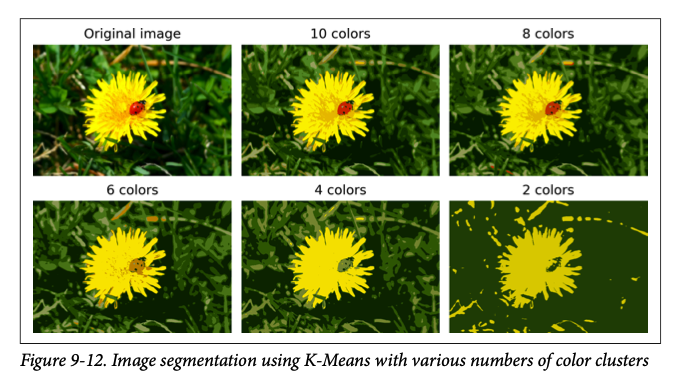## Section8Clustering

We are finally getting another example of unsupervised learning!!! Remember that unsupervised learning involves giving unlabeled data to an algorithm and asking the algorithm to find some structure or patterns in the data. In our previous example of unsupervised learning, PCA, we constructed an algorithm to find the structure of a lower dimensional space in the data. For clustering, we want to construct an algorithm to group similar instances into clusters.

In this course, we are focusing primarily on supervised learning. Its the traditional starting place, and many of the most exciting current applications are based on these techniques. However, supervised learning requires labeled data. The vast majority of available data is unlabeled and labeling data is an expensive process because it almost always require human time to label the data. Facebook artificial intelligence Chief, Yann LeCun, gave a now famous analogy at NIPS 2016 (Conference on Neural Information Processing Systems).

If intelligence was a cake, unsupervised learning would be the cake, supervised learning would be the icing on the cake, and reinforcement learning would be the cherry on the cake
at NIPS 2016 (Conference on Neural Information Processing Systems). An image of one of his slides is below.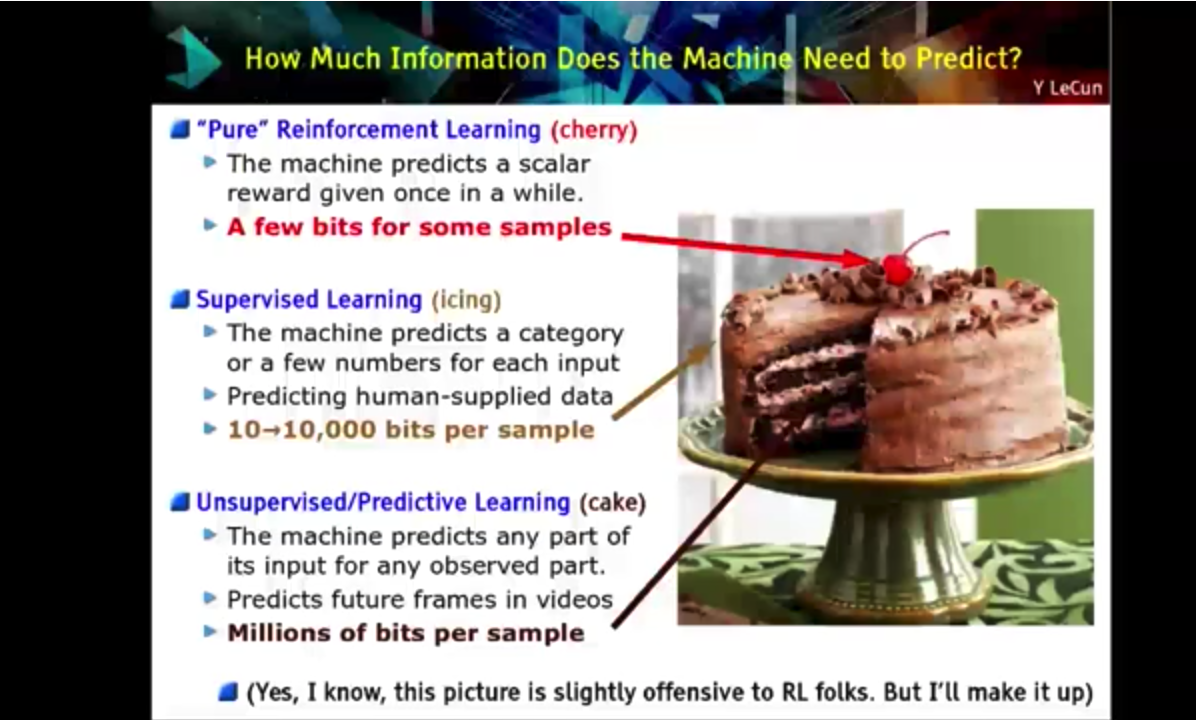The entire talk is available on You-Tube if you want to check it out.
The cake reference and above image can be found at time stamp 19:26. He more recently (2019) updated unsupervised learning to self-supervised learning. But the idea is the same; unsupervised learning is really important in machine learning!

Clustering has a wide array of uses in data analysis. Examples include:

1. Market Segmentation: Identify market segments to better design products to appeal to similar groups of customers.
2. Anomaly Detection: Identify points that are far away from any cluster. Can be used to detect fraud or manufacturing defects.
3. Search Engines: Identify similar pages/images/etc to return results for a search.
4. Image Segmentation: Cluster pixels in an image according to their color to reduce the number of colors in an image. Often useful in detection and tracking systems.
5. Data Analysis: One technique for data analysis on large data sets is to run a clustering algorithm and analyze each cluster separately.
6. Social Network Analysis: Analyze clusters of friends.
7. Data Center Analysis: Organize computer clusters/data centers based on which computers/servers talk to each other most frequently.
8. Astronomical Data Analysis: Analyze the formation of galaxies.
9. Semi-Supervised Learning: If you have data that you want labeled for supervised learning, but only have a small amount of labeled data, you can apply clustering and propagate the labels to all points in those clusters.

### Subsection8.1K-means Clustering

There are many different clustering algorithms but we will focus on the K-means algorithm which is by far the most popular and widely used. K-Means is a simple, iterative algorithm that can cluster a dataset quickly and efficiently, often in just a few iterations. It was proposed by Stuart Lloyd at Bell Labs in 1957 as a technique for pulse-code modulation, but not published outside of the company until 1982. In 1965, Edward W. Forgy published a very similar algorithm.

The idea behind the K-means algorithm is a simple iterative process. The number of clusters, $K\text{,}$ must be specified.

1. Step 1

1. Assign a centroid for each of the $K$ clusters randomly.
2. Compute the distance between each point and each of the $K$ centroids.
3. Assign points to a cluster based on the nearest centroid. (There is a choice to make if there is a tie. We will assign these randomly.)
2. Step 2

1. Assign a centroid for each of the $K$ clusters by computing the mean value of all points in the cluster.
2. Compute the distance between each point and each of the $K$ centroids.
3. Assign points to a cluster based on the nearest centroid.
3. Step 3 - Step $n\text{.}$ Repeat step 2 until the centroids converge to a stable value.

###### Example8.1.

Suppose we have a data set with two features and six points and we want to group the data into two clusters.

 $x_1$ $x_2$ 1.1 2.0 2.1 0.0 4.1 1.0 2.9 4.0 4.9 2.0 1.1 4.0 4.1 5.0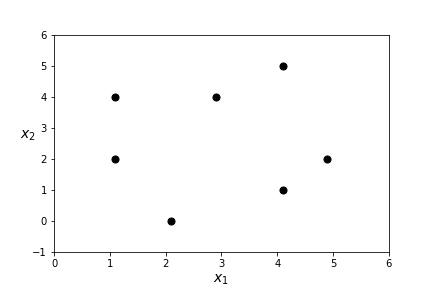###### Question8.2.
What two clusters do you see?

Let's see what clusters K-means finds!

1. Step 1

1. Assign a centroid for each of the $K$ clusters randomly. Pick $c_1=(3,3)$ and $c_1=(4,4)$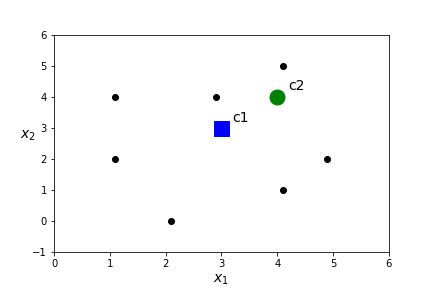2. Compute the distance between each point and each of the $K$ centroids. Assign points to a cluster based on the nearest centroid.
 $x$ dist(x,c1) dist(x,c2) closest centroid (1.1,2.0) 2.15 3.52 c1 (2.1,0.0) 3.13 4.43 c1 (4.1,1.0) 2.28 3.00 c1 (2.9,4.0) 1.00 1.10 c1 (4.9,2.0) 2.15 2.19 c1 (1.1,4.0) 2.15 2.90 c1 (4.1,5.0) 2.28 1.00 c2
3. Assign points to a cluster based on the nearest centroid.2. Step 2

1. Assign a centroid for each of the $K$ clusters by computing the mean value of all points in the cluster. In this case
\begin{equation*} c_1=\frac{(1.1,2)+(2.1,0)+(4.1,1)+ (2.9,4)+(4.9,2)+(1.1,4)}{6}= (2.7,2.17) \end{equation*}
\begin{equation*} c_2=(4.1,5). \end{equation*}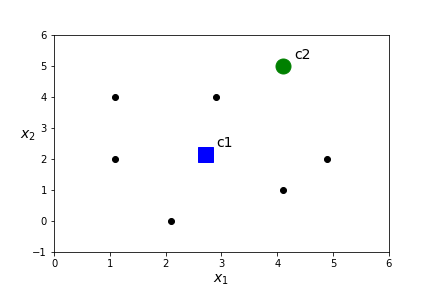2. Compute the distance between each point and each of the $K$ centroids.
 $x$ dist(x,c1) dist(x,c2) closest centroid (1.1,2.0) 1.61 4.24 c1 (2.1,0.0) 2.25 5.39 c1 (4.1,1.0) 1.82 4.00 c1 (2.9,4.0) 1.84 1.56 c2 (4.9,2.0) 2.21 3.10 c1 (1.1,4.0) 2.43 3.16 c1 (4.1,5.0) 3.16 0.00 c2
3. Assign points to a cluster based on the nearest centroid.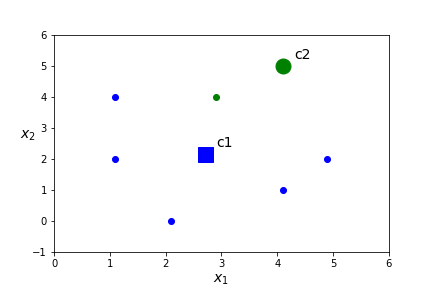3. Step 3

1. Assign a centroid for each of the $K$ clusters by computing the mean value of all points in the cluster. In this case
\begin{equation*} c_1=\frac{(1.1,2)+(2.1,0)+(4.1,1)+(4.9,2)+(1.1,4)}{5}= (2.66,1.8) \end{equation*}
\begin{equation*} c_2=\frac{(4.1,5)+ (2.9,4)}{2}=(3.5,4.5). \end{equation*}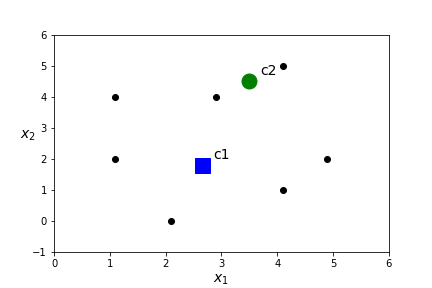2. Compute the distance between each point and each of the $K$ centroids.
 $x$ dist(x,c1) dist(x,c2) closest centroid (1.1,2.0) 1.57 3.47 c1 (2.1,0.0) 1.89 4.71 c1 (4.1,1.0) 1.65 3.55 c1 (2.9,4.0) 2.21 0.78 c2 (4.9,2.0) 2.25 2.87 c1 (1.1,4.0) 2.70 2.45 c2 (4.1,5.0) 3.51 0.78 c2
3. Assign points to a cluster based on the nearest centroid.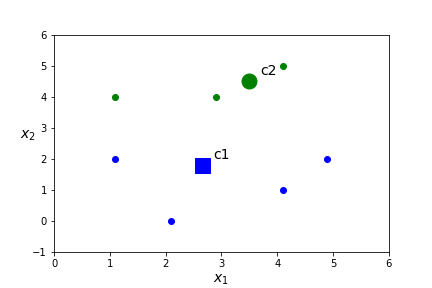4. Step 4

1. Assign a centroid for each of the $K$ clusters by computing the mean value of all points in the cluster. In this case
\begin{equation*} c_1=\frac{(1.1,2)+(2.1,0)+(4.1,1)+(4.9,2)}{4}= (3.05,1.25) \end{equation*}
\begin{equation*} c_2=\frac{(4.1,5)+ (2.9,4)+(1.1,4)}{3}=(2.7,4.33). \end{equation*}2. Compute the distance between each point and each of the $K$ centroids.
 $x$ dist(x,c1) dist(x,c2) closest centroid (1.1,2.0) 2.09 2.83 c1 (2.1,0.0) 1.57 4.37 c1 (4.1,1.0) 1.08 3.62 c1 (2.9,4.0) 2.75 0.39 c2 (4.9,2.0) 2.00 3.21 c1 (1.1,4.0) 3.37 1.63 c2 (4.1,5.0) 3.89 1.55 c2
3. Assign points to a cluster based on the nearest centroid.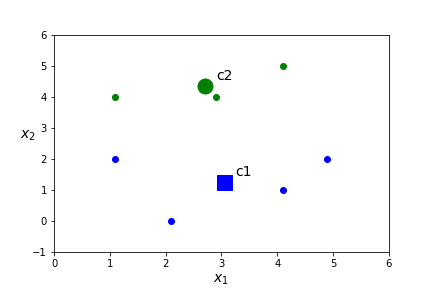###### Question8.3.
What will happen in Step 5? Answer
Nothing will change. The clusters have stabilized and the centers will not change if we continue the process. So we are done!

###### Question8.4.
What do you think of the clusters K-means found? Answer
In this case, it is unclear if these are the best clusters for the data. The data isn't very cluster-y.

###### Question8.5.
Try some different values for centers in the code below and see if you can create different clusters.

Hint

It is quite easy to produce different clusters with different initial centroids. Try cent=np.array([[0,2],[5,2]]) or cent=np.array([[0,0],[4,4]])

We can see in the code that it is easy to use sklearn to invoke KMeans. We need

from sklearn.cluster import KMeans
kmeans=KMeans(n_clusters=2,init=cent,n_init=1)


The full list of parameters are

KMeans(algorithm='auto', copy_x=True, init='k-means++', max_iter=300,
n_clusters=8, n_init=10, n_jobs=None, precompute_distances='auto',
random_state=None, tol=0.0001, verbose=0)


The main parameter to explore is n_clusters. In some cases there is an obvious number of clusters based on the purpose of the model, but usually this is something we want to explore.

n_init=10 is the number of times the program will be run with different randomly chosen initial centers. As we have seen, this is quite important as KMeans is very sensitive to this initial choice of centers.

There are a few optimizations for KMeans that we will discuss in the next section. There are better strategies for picking initial centers other than picking random centers. (This corresponds to the init parameter.) It can be a lot of work to compute all the distances and there are some optimizations of that process related to choices in algorithm and precompute_distances.

Important attribues already used in the above code are  cluster_centers_  and  labels_ .

###### Question8.6.
Below are four of the different clusters that are possible based on different initial centroids. Which one do you think is best? What should best mean?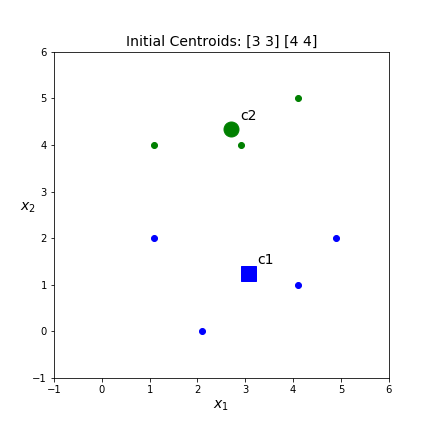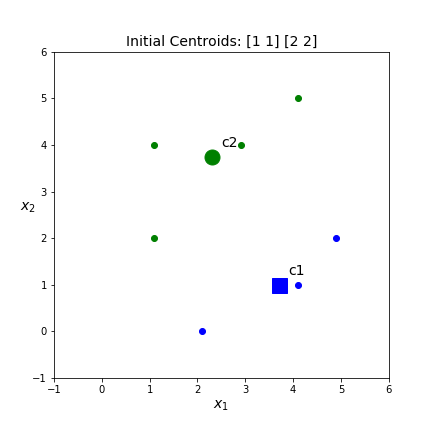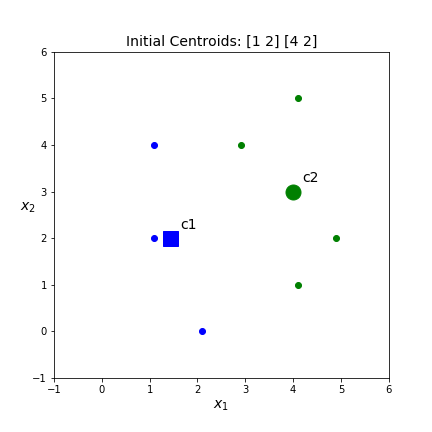Since we are clustering based on the distance to the centroid we would like the set of clusters that have the smallest distance between points and centroids. To simplify a little we will calculate the sum of the squared distances between points and their centroid. For example, in case 1: The cluster centers are
\begin{equation*} c_1=(3.05,1.25) \end{equation*}
and
\begin{equation*} c_2=(2.7,4.33). \end{equation*}
The points in cluster1 with centroid $c_1=(3.05,1.25)$ are $(1.1,2),(2.1,0),(4.1,1),(4.9,2)\text{.}$ The points in cluster2 with centroid $c_2=(2.7,4.33)$ are $(4.1,5), (2.9,4),(1.1,4)\text{.}$ The sum of the squared distances are
\begin{equation*} \| (1.1,2)-(3.05,1.25) \|^2 + \| (2.1,0)-(3.05,1.25) \|^2 \\ + \| (4.1,1)-(3.05,1.25) \|^2 + \| (4.9,2)-(3.05,1.25) \|^2 \\ + \| (4.1,5)-(2.7,4.33)\|^2 + \| (2.9,4)-(2.7,4.33)\|^2\\ + \| (1.1,4)-(2.7,4.33)\|^2 \\ = 4.36+2.47+1.16+3.99+2.41+0.15+2.67 \\ = 17.21 \end{equation*}
This can also be calculated as kmeans.inertia_ . This is the score KMeans uses to determine which of the different sets of clusters is best. The inertia scores for the above clusters are 17.21, 22.07, 17.39, 20.71 respectively. Thus, the top left set of clusters is the best. However, both left hand sets of clusters are pretty similar.

### Subsection8.2Optimizing KMeans

The optimization objective in KMeans clustering is to minimize the sum of squared distances between each point and its closest centroid. To write this as a formula let $c_j$ denote the $j$th cluster centroid. (In sklearn, kmeans.cluster_centers_[j].) Let $L_i$ denote the index of the cluster that $x^i$ is assigned to (In sklearn, kmeans.labels_[i].) Then the cluster centroid that is closest to $x^i$ is given by $c_{L_i}\text{.}$ (In sklearn, kmeans.cluster_centers_[kmeans.labels_[i]]). Thus our optimization objective $J$ is a function of both the centroids and the cluster assignments and is given by

\begin{equation*} J (\text{centroids, cluster assignments}) = \sum_{i=1} ^m \| x^i - c_{L_i} \|^2. \end{equation*}

(In sklearn, kmeans.inertia_).

###### Question8.7.
How do the steps in KMeans clustering optimize this function? Answer

Essentially we have two steps that we alternate between

Step A: For fixed centroids, assign points to a cluster based on the closest centroid. This assignment of points will minimize the sum of squared distances between points and a fixed set of centroids. (Minimizes J as we vary cluster assignment, but fix centroids.)

Step B: For fixed cluster assignments, change the centroid to the mean of all points in the cluster. This is the location that will minimize the sum of distances between points and centroid for a fixed cluster assignment. (Minimizes $J$ as we vary centroids, but fix cluster assignment.)

Alternating between these two steps will minimize $J$ relative to both centroids and cluster assignments.

###### Question8.8.
What should happen to $J$ as we iterate the algorithm for a fixed number of clusters? Can $J$ ever increase? Can $J$ remain constant? Answer
$J$ can never increase. Each step moves to a lower value of $J$ or remains the same. Thus, $J$ must decrease until it converges. But it can remain constant!

###### Question8.9.
What should happen to $J$ as we increase the number of clusters $K\text{?}$ Can $J$ ever increase? Can $J$ remain constant? Could $J=0\text{?}$ Answer
$J$ can not increase. As we add more centorids the distance between points and centroids must decrease. The only way $J=0$ is if the distance between all points and centroids is 0. This can only happen if every point is its own centroid.

We've seen how much impact the starting choice of centroids can have to the final set of clusters. It is entirely possible that this algorithm can get stuck at local minimum for $J\text{.}$ One solution is choose multiple different random initializers and keep the one with the lowest cost. The default option is to choose ten different initial centroids. But there are some other options that will help the algorithm converge more quickly. n_init=10

###### Question8.10.
Should we ever pick two initial centroids to be the same? What about just close? Answer
We definitely do not want to pick the centroids to be the same. Essentially we lose cluster. We do not want them to be close either for best results. In fact, trying to pick initial centroids that are far apart is helpful.

Here are three options for picking initial centroids:

1. Pick $k$ random centroids. We did this in the example, but it isn't recommended in practice.
2. Pick k distinct points from the data set. (Randomly, but points are chosen randomly from data set rather than randomly from a given distribution.) init='random' implements this choice.
3. Pick one centroid at random from the data set. Then picks the next according to a probability distribution which encourages the next centroid to be far away from the centroids selected so far. init='k-means++' is the default setting.

How should we choose the number of clusters?

1. There may be a goal of your data set that specifies the best number of clusters. You want to design T-shirts and will have 3 sizes, small, medium, large. You can identify three clusters of clothing proportions in your data.
2. In many cases, there is not a right answer.
3. One technique is to apply the "elbow technique". Plot J versus the number of clusters. J will keep decreasing as K increases, but there may be an "elbow" where it appears increasing L does not result in a substantial lowering of J.

Much of the work in KMeans involves computing distances. This can get computationally overwhelming. There is a technique called the Elkan technique which uses the triangle inequality to keep track of lower and upper bounds for distances between points and centroids. Whether to use the Elkan technique or not, really depends on the type of data you have. It does not support sparse data. However algorithm='auto' will take care of making this decision for you.

###### Question8.11.
What is the triangle inequality? Answer
In a triangle the sum of the lengths of two sides of the triangle must be less than the length of the third side.\begin{equation*} AC \leq AB +BC \end{equation*}

Even with all these speed-ups, if the data set is really large, then KMeans will be slow. For large data sets it is much better to perform KMeans on subsets of the data (minibatches) and then combine the results. This can be three to four times faster and can also handle a dataset that doesn't fit in memory. Of course, it will perform slightly worse, especially as K increases. It will also not have a strictly decreasing J.

from sklearn.cluster import MiniBatchKMeans
batchkmeans=MiniBatchKMeans(n_clusters=10)
batchkmeans.fit(X_train)


The full list of parameters:

MiniBatchKMeans(batch_size=100, compute_labels=True, init='k-means++',
init_size=None, max_iter=100, max_no_improvement=10,
n_clusters=10, n_init=3, random_state=None,
reassignment_ratio=0.01, tol=0.0, verbose=0)


The main new parameter is to specify batch size.

###### Question8.12.
When do we want a larger batch size and when do we want a smaller batch size? Answer
A smaller batch size will be faster and a larger batch size will have a smaller cost.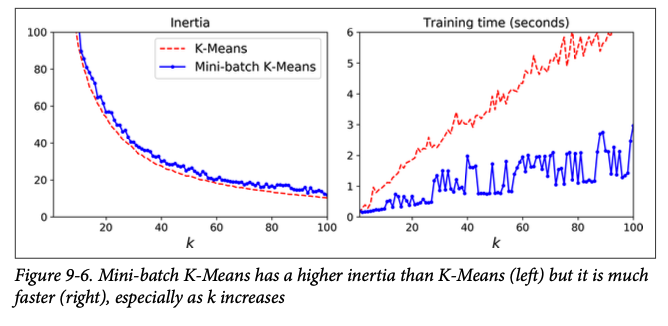KMeans has many merits! Its fast and tends to work well on large data sets (especially with minibatch KMeans.) But the size, density and shape of clusters strongly impact KMeans. KMeans works best if clusters have similar size and density and a spherical shape.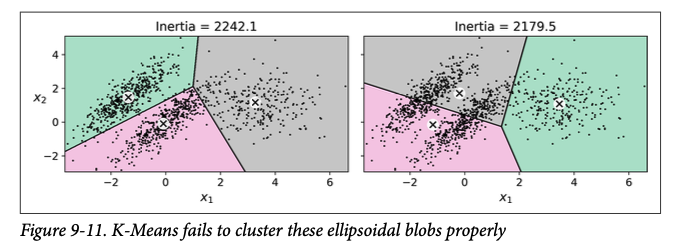It is really important to scale features first as it will make shapes more spherical and improve performance.

There are other methods of clustering that will work better for some types of data, but KMeans works surprisingly well in a wide variety of cases. We will conclude with an example of color segmentation. For an image we will cluster colors to reduce the number of colors used in the image, possibly for compression or some type of image detection/analysis. It is surprising how few colors we need to fully represent the image!